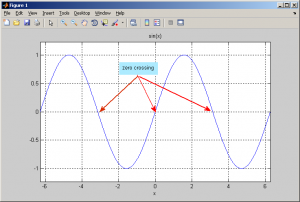# C/C++ Zero Crossing code

Zero-crossing is a commonly used term in electronics, mathematics, and image processing. In mathematical terms, a “zero-crossing” is a point where the sign of a function changes (e.g. from positive to negative), represented by a crossing of the axis (zero value) in the graph of the function. This is picture describe zero crossing location :I have some of data and want to get zero crossing from that data. So, I try to create C/C++ zero crossing code to solve that problem. This my simple C/C++ zero crossing code.

```/* zero crossing function */
/* data = input array */
/* zerCross = output zero crossing array */
void zeroCrossing(float *data, float *zerCross, int nx)
{
int i;
bool sign1, sign2;

memset(zerCross, 0, nx*sizeof(float));
for(i=0; i<nx-1; i++)     /* loop over data  */
{
sign1 = getSign(data[i]);
sign2 = getSign(data[i+1]);
if(sign1!=sign2)  /* set zero crossing location */
zerCross[i+1] = 1;
}
}

/* get sign of number */
bool getSign(float data)
{
if(data>0)      /* positif data */
return (1);
else            /* negatif data */
return (0);
}```

Output from this C/C++ zero crossing function is array with value 0 and 1. Value 1 is location of zero crossing data.

Source :
http://en.wikipedia.org/wiki/Zero_crossing